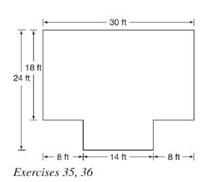Chapter 8.2, Problem 36EElementary Geometry For College St...

7th Edition
Alexander + 2 others
ISBN: 9781337614085

Solutions

Chapter
SectionElementary Geometry For College St...

7th Edition
Alexander + 2 others
ISBN: 9781337614085
Textbook Problem

Find the perimeter of the room in Exercise 35.To determine

To Find:

Perimeter of the room provided the floor plan.

Explanation

Formula Used:

The perimeter of a polygon is the sum of the lengths of all sides of the polygon.

Calculation:

Let the vertices of the provided octagon be A, B,C, D, E, F, G and H.

It is given that AB=18 ft, BC=FG=8 ft, DE=14 ft, and AH=30 ft

Still sussing out bartleby?

Check out a sample textbook solution.

See a sample solution

The Solution to Your Study Problems

Bartleby provides explanations to thousands of textbook problems written by our experts, many with advanced degrees!

Get Started

If f is periodic, then f is periodic.

Single Variable Calculus: Early Transcendentals, Volume I

A sample with a mean of M = 8 has X = 56. How many scores are in the sample?

Essentials of Statistics for The Behavioral Sciences (MindTap Course List)

limx0+(1+x)1/x= a) e b) e c) 1e d) 1e

Study Guide for Stewart's Single Variable Calculus: Early Transcendentals, 8th